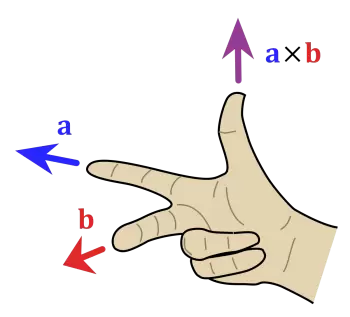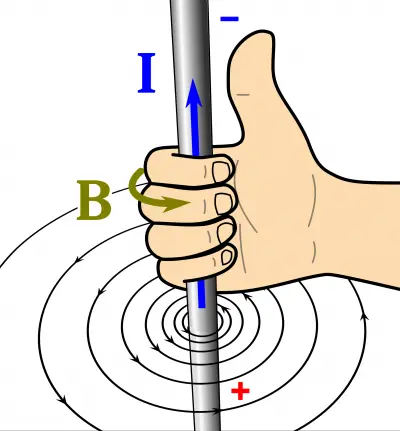# What Is the Right Hand Rule?

The right-hand rule is a convention that is used to know the direction of specific vectors in the field of mathematics and physics. This rule allows knowing the direction of the vector that results from a vector product.In the product of vectors “a” and “b,” we represent vector a by the index finger and vector b by the middle finger. The thumb points to the direction of the resulting vector, as shown in the figure.

In electricity and magnetism, we can know the direction of a charge in a magnetic field through the law of the hand grip rule. To determine the direction, we place the right hand's thumb in the same direction as the electric current passing - from positive to negative. Then, if we bend the remaining fingers of the hand, they indicate the direction of the magnetic field lines.There is also another version known as the three-finger rule. In this case, the right hand's thumb indicates the speed of the movement of the conductor, the index finger points to the direction of the induction of the magnetic field. Lastly, the bent middle finger represents the return of the induced current.

John Ambrose Fleming was the inventor of this rule to determine the direction of the magnetic field that produces moving charges. For that reason, this rule is also called the fleming right-hand rule.

## Right-hand Rule Uses

The rule is used to determine the direction of the vector resulting from the cross-product of two vectors. In physics, it is used to know the direction of many physical quantities. Here is a list of applications of the rule in physics:

• The angular speed of a rotating object and the rotation speed of any point on that object

• The mechanical moment, the force that causes it, and the location of the point of the force exerted.

• A magnetic field, the location of the point where it is determined, and the electric current (or charged particle in the electric flow) that causes it.

• A magnetic field in a spiral of wire and an electric current in the wire

• The force that a magnetic field exerts on a given object, the object's speed, and the magnetic field itself.

• The current induced moving in a magnetic field.

• Fleming's left-hand rule can be used to remember the direction of the Lorentz force.

• It is used to represent a Cartesian reference system.

Author:

Published: January 14, 2022
Last review: January 14, 2022